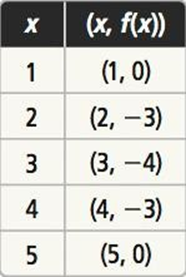Maths-
General
Easy

Question

# Compare each function to f, shown in the table. Which function has lesser minimum value? Explainh(x) = x2 + x – 3.5

## The correct answer is: -3.75

### We have given two functions f(x) and h(x).h(x) = x2 + x – 3.5For f(x) , minimum value of the function will be the y-coordinate of the given point which has minimum values(1,0) , (2,-3) , (3,-4) , (4,-3) (5,0)In the given points minimum value of is (3,-4)So, the ,minimum value of f(x) is -4.In h(x) = x2 + x – 3.5,  a= 1, b= 1, and c = -3.5. So, the equation for the axis of symmetry is given byx = −(1)/2(1)x = -1/2x = -0.5The equation of the axis of symmetry for h(x) = x2 + x – 3.5 is x = -0.5.The x coordinate of the vertex is the same:h =-0.5The y coordinate of the vertex is :k = f(h)k = h2 + h – 3.5k = (-0.5)2 + (-0.5) – 3.5k = 0.25 – 0.5 – 3.5k = -3.75Therefore, the vertex is (-0.5 , -3.75)The minimum value of g(x) will be the y-coordinate of vertex = -3.75#### With Turito Foundation.#### Get an Expert Advice From Turito.